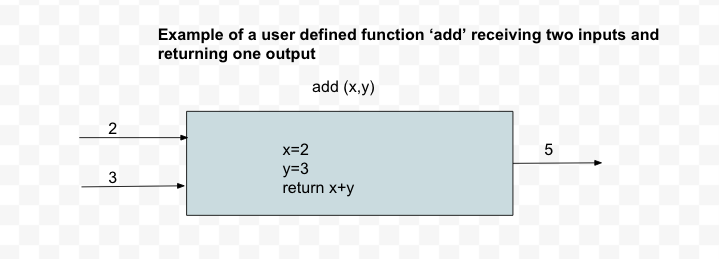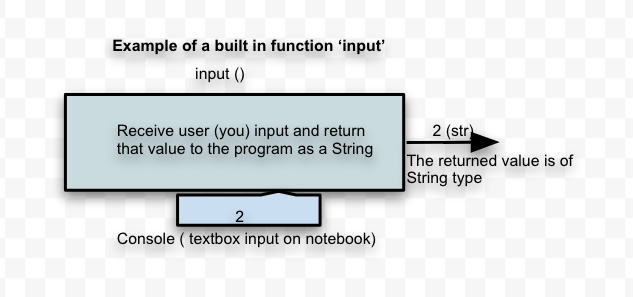# Function - Intro

You will deep dive into defining functions later. However, for now we will understand the fundamental concept of a function.

A function is a set of statements, represented by a name, that take zero to more inputs, do some computation and produces zero to more outputs. You send inputs (any expression, variable or literal values can be an input) to a function between a pair of parenthesis(). If the function returns something, then, you receive that, by typically declaring a variable to hold that value, or pass it as an input to another function.

Here is an example of an imaginary user defined function named 'add' which receives two inputs; 2 and 3 in this example and it returns 5 when it is invoked.The python code for invoking the function 'add' is shown below. In this example you are only invoking this function. But before you can invoke a function, it should be defined elsewhere in the program and you will learn more on that later. But for now we understand how to invoke the function once it is defined elsewhere.

``````
print(b)
``````

Output:
5

# Built In Functions

Python already provides us with several functions that are already defined by the Python engineers and we just get to invoke them when ever we need. These are called 'built-in' functions as we are not defining them ourselves, but instead just using (invoking) them.

Let us now understand one very handy 'built-in' function called 'input', which helps you to receive values from the user and another built-in function called 'int' which converts a given string data into an integer data type.

#### input function

To receive any input from the user when the program is in execution, you use the input function.In the above diagram there is no input given to the function 'input' inside the parenthesis. Note we are using the word 'input' for two different entities here. One is the input to the function and the other, the function itself is called 'input'. Once you run this code, the program stops its execution and waits as it expects you to input a value on the console if you are running from the command line. But in our case since we are running on the Jupyter notebook, it will open a textbox and expects us to input value inside the textbox so that the program can receive it.

The code for this would be

``````a = input()
a
``````

When you run the above code, be sure to input some value in the textbox that shows up and what ever is input into the textbox, will be displayed from the program.

But you can also send a string inside the parenthesis which will be displayed to the user. Here is that example:

``````name = input("Please enter your name ")
print('Welcome', name)
type(name)
``````

When you run the above code, a message 'Please enter your name' is first displayed and then the Python interpreter waits for you to input some value which will be received as a String. A prompt appears on the browser where you need to input the value.

Note: The value that is received in the textbox is stored in the variable 'name' which is assigned a 'str' type as can be seen from the output of the above program.

#### int function

Another convenient built-in function is 'int' which helps you convert a given String to Integer type as long as the given String is a literal value of an integer. Here is an example

``````a = '10'
b = int(a)
type(a), type(b)
``````

Output:
(str, int)

So in the above code, the literal value that is assigned to 'a' is a String due to the value 10 being enclosed in single quotes. This value is then passed on to the 'int' function which will convert the data type from 'str' to 'int' as can be seen in the output.

#### 'input' and 'int' Function Chaining

Anything that is received from the 'input' function is received as 'str' or String type only by default. Even if you input a number or a decimal, it is received as a String in the program. To convert this String to an integer, you use the 'int' built-in function. And to save the intermediate results, you can chain the two functions as shown below.

``````age = int(input("Please enter your age "))
print(age)
type(age)
``````

Output: what ever number you entered in the input box.

When you run the above example, you are again prompted to input a value. By default the value that is entered by the user is read by input function as a String. When this value is then passed to the int() function, the given string is converted into an integer value and returned from this function, which is then saved in the variable 'age'. If you do not input a number in the console, then a ValueError is thrown as int function cannot convert any other input to integer other than any value which is a number without a decimal point. Note that even though you input a number from the keyboard, input function still returns that number as a string and the int function converts that string to a number.

If you had not chained the two functions together then you would be declaring additional variables to hold the intermediate results and here is the long program which does just that:

``````age_str = input("Please enter your age ")
age = int(age_str)
type(age), type(age_str)
``````

Output:
(int, str)

In this long program, we introduced an additional variable age_str which keeps the value returns from 'input' and then send this variable as input to the 'int' program. This way it not only introduces additional unnecessary variables , which occupy memory space, but also increases the number of lines to code to accomplish the same functionality. Instead function chaining is more appropriate some simple functions like these. However, chaining too many functions can become very hard to understand the code. Easy readability of the code trumps over code efficiency and hence you should be prudent when to use and when not to use chaining multiple functions.

#### Other Built-In Functions

To receive a number with a decimal point, you would use float instead of int in the above program. To receive a boolean value, you could use 'bool'

You can use eval() function to evaluate a string representation of a Python statement. Here are some examples:

``````eval('10+30')
eval("print('hello')")
``````

Python provides a number of such built in functions and here link to the official documentation: https://docs.python.org/3/library/functions.html

##### More on 'print' function

'print' function is something that a newbie will use liberally to understand the concepts of programming. Let us understand a few variations of using this 'print' built-in function.

Multiple Arguments

You can print more than one variable or literal or expressions which lead to a literal by using a comma (,) separator to and send in the list of arguments to the function:

``````
a = 10
b = 15.0
c = True
d = 'hey'
print("Numbers", a, b, a * b, c, d)
``````

Output:
Numbers 10 15.0 150.0 True hey

In this example, the print function received 6 arguments and each one evaluates to any data type which is converted to a String and printed on the console. You can send as many arguments as you want.

Keyword Argument 'sep'

By default the separator between the arguments is a space. But you can change this separator by providing a 'sep' keyword with a value. Here is an example:

``````
a = 10
b = 15
print("Numbers", a, b, a * b, sep = ",")
``````

Output:
Numbers,10,15,150

Arguments like 'sep' which are in the form of 'name=value' T are called keyword arguments.

Keyword Argument 'end'

By default each print is printed on a new line. Here is an example

``````
print(10)
print(20)
``````

Output:
10
20

However, you can remove this newline character by sending in the keyword 'end' with the required end literal. If nothing is given, it defaults to newline

``````
print(10, end = "")
print(20)
``````

Output:
1020

#### Comment lines

Sometimes you want to insert a few lines in the code block which are meant for explaining your logic and not for the Python interpreter to execute those lines. Comments are used to explain more on the workings of the program, which is not obvious from reading the code itself! Although all detailed explanations and documentation should go in the 'Text' block of your Jupyter notebook, you can also add comment statements in your code block.

You can create a comment statement by starting the text with a #, and Python will ignore those lines.

Example:

``````
# This is a comment line and is not executed by Python
print("Hello!")
``````

You can also place a comment after a regular statement. Here is an example:

``````print("Hello!")  # A comment can be here too
``````

A working code can be commented out so that it does not execute. Here is an example:

``````print("Hello!")
# print("world")
``````

#### Multiline comment

When your comment spans multiple lines, instead of commenting out every line, you can use multiline string literals which will ignored by Python.

Here is an example:

``````''' A mutliline comment typically
used for documentation on help '''

print("hello")
``````

Note the string literal is not assigned to any variable.

You can also use three double quotes instead of three single quotes to get the same results.